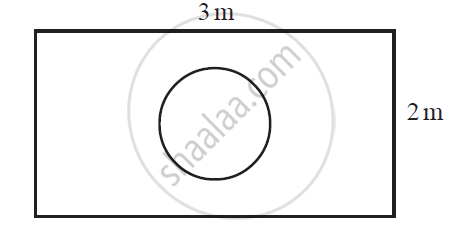# Suppose you drop a die at random on the rectangular region shown in the given figure. What is the probability that it will land inside the circle with diameter 1m? - Mathematics

Sum

Suppose you drop a die at random on the rectangular region shown in the given figure. What is the probability that it will land inside the circle with diameter 1m?#### Solution

Area of rectangle = l × = 3 × 2 = 6 m2

Area of circle (of diameter 1 m)  = pir^2= pi((1pi)/2)^2 = pi/4 m^2

P (die will land inside the circle) = (pi/4)/6= pi/24

Concept: Probability - A Theoretical Approach
Is there an error in this question or solution?
Chapter 15: Probability - Exercise 15.1 [Page 310]

#### APPEARS IN

NCERT Class 10 Maths
Chapter 15 Probability
Exercise 15.1 | Q 20 | Page 310

Share